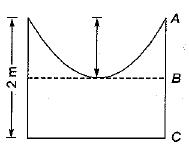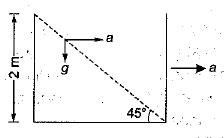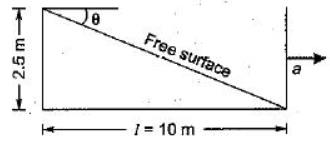Courses

# Test: Liquids In Relative Equilibrium

## 9 Questions MCQ Test Topicwise Question Bank for GATE Civil Engineering | Test: Liquids In Relative Equilibrium

Description
This mock test of Test: Liquids In Relative Equilibrium for Civil Engineering (CE) helps you for every Civil Engineering (CE) entrance exam. This contains 9 Multiple Choice Questions for Civil Engineering (CE) Test: Liquids In Relative Equilibrium (mcq) to study with solutions a complete question bank. The solved questions answers in this Test: Liquids In Relative Equilibrium quiz give you a good mix of easy questions and tough questions. Civil Engineering (CE) students definitely take this Test: Liquids In Relative Equilibrium exercise for a better result in the exam. You can find other Test: Liquids In Relative Equilibrium extra questions, long questions & short questions for Civil Engineering (CE) on EduRev as well by searching above.
QUESTION: 1

### An oil tanker is partially filled and is then moved in translation at uniform acceleration. The free surface of oil then

Solution:

In this case the surface of constant pressure is a downward sloping plane surface. The free surface of the liquid is a surface of cohstant pressure, because the pressure at the free surface is equal to atmospheric pressure which is constant. As such under the effect of the horizontal acceleration the free surface of the liquid will become a downward sloping inclined plane, with the liquid rising at the back end and the liquid falling at the front end.

QUESTION: 2

### The free surface of a liquid partially filled in a cylindrical container when subjected to uniform rotation assumes a shape which can be represented by

Solution:

The shape of the free surface is paraboloid.

QUESTION: 3

### A closed tank containing water to a depth of 1.5 m falls vertically with an acceleration of 2 m/s2. What is the pressure at the bottom of the tank?

Solution:

Pressure at bottom of tank
= p (g - a) h = 1000 x (9.81 - 2) x 1.5
= 11.7 kN/m2

QUESTION: 4

A cylindrical vessel with a constant plane area of 1 m2 is rotated about its vertical axis such that the liquid inside the vessel is about to spill. If the height of the vessel is 2 m and the height of the paraboloid is 1 m, then the volume (in m3) of the liquid in the vessel will be

Solution:height of paraboloid = Z = 1 m = r2w2/2g
VoI. of water = VAB + VBC
VAB = Volume of paraboloid
= 1/2 volume of circumscribing cylinder
VBC = Cross- sectional area x 1
1/2 x 1 x 1 = 0.5 m3
Total volume of water = 1.5 m3

QUESTION: 5

A right circular cylinder, open at the top is filled with liquid, sp. gr. 1.2 and rotated about its vertical axis at such speed that half the liquid spills out. The pressure at the centre of the bottom is

Solution:

After half the water has spilled out the vertex of the paraboloid will touch the bottom of cylinder thus pressure will be zero.

QUESTION: 6

An open rectangular wagon 5 m long partly filled with water is subjected to an acceleration of 1.96 m/s2. For bn spilling of water the level below the open top upto which water may be filled is

Solution:
QUESTION: 7

When a cylindrical vessel containing liquid is revolved about its vertical axis at a constant angular velocity, the pressure

Solution:

Variation in pressure in horizontal direction in a rotating cylindrical vessel containing fluid is given by,
∂P/∂r = pw2r

QUESTION: 8

An open cubical tank of 2 m side is filled with water. If the tank is accelerated horizontally with an acceleration such that half of the water spills out, then the acceleration is equal to

Solution:⇒ tan 45º = a/g ⇒  a = g

QUESTION: 9

A 3 m wide, 2.5 m deep, 10 m long tank, open at the top, has oil standing to 1 m depth. The maximum horizontal acceleration that can be given to the tank without spilling the oil will nearly be

Solution:

In the case of maximum acceleration, the free surface will be such that one end will have 2.5m depth and the other will have zero depth. Equating volume contained by free surface to earlier volume;
l x 2.5/2 x 3 = 3 x 10 x 1
I = 20/2.5 = 8m
tanθ = 2.5/8 = a/g
a = 0.31g